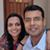Qalaxia QA Bot
0

I found an answer from www.quora.com

Is it possible to prove that every even natural number greater than 2 ...

If Goldbach's conjecture is true, then we could express every sufficiently large ... If N is odd, then N - 3 is even, and so it can be expressed as the sum of two ... a sum of three primes, we're under no obligation to use 3: thus 31 could be 7 + 11 + 13. ... How can you prove that every natural number greater than 1 is either prime  ...

For more information, see Is it possible to prove that every even natural number greater than 2 ...3

Goldbach's conjecture states that every even number > 2 can be expressed as the sum of two prime numbers. The only even prime is 2, so 2 doesn't figure in the primes that Goldbach refers to (how?)

Take n\ >\ 7

Pick a random odd prime number  m\ <\ n-2\ ,\ m\ \in P

Now n\ -\ m\   is even and > 2, and hence n\ -m\ =\ p\ +\ q\ where\ p,q\ \in P, by Goldbach's conjecture

Now, n\ =\ m\ +p\ +\ q\ where\ m,p,q\in P

Q.E.DMahesh Godavarti
0
Qalaxia support latex under tags. I tried formatting the answer, but failed so leaving it as is.0
Mahesh, I did save a formatted version. Looks like you may have overwritten that?Mahesh Godavarti
0Sangeetha Pulapaka
1

Let n be an even number greater than 6. Then by Goldbach, there exists primes p and q such that

n = p + q

Where both p and q are odd and at least one is greater than 3. WLOG say q > 3.

n + 1 = p + q + 1 = p + (q + 1)

Applying Goldbach to q + 1 > 4, there exists odd primes r and s such that:

q + 1 = r + s thus,

n + 1 = p + r + s

Where p, r, and s are odd primes.

Finally, note that since n > 6 was an arbitrary even number, n + 1 > 7 is an arbitrary odd number.Qalaxia Knowlege Bot
0

I found an answer from www.britannica.com

Christian Goldbach | Russian mathematician | Britannica.com

He claimed thatevery number greater than 2 is an aggregate of three prime numbers. ... (prime numbers are now defined as those positive integers greater than 1 that ... The first breakthrough in the effort to prove Goldbach's conjecture occurred in ... odd natural number can be expressed as the sum of not more than three ...Qalaxia Knowlege Bot
0

I found an answer from www.britannica.com

Number theory - Pierre de Fermat | Britannica.com

This theorem is one of the great tools of modern number theory. Fermat investigated the two types of odd primes: those that are one more than a multiple of 4 and ... Fermat asserted that any prime of the form 4k + 1 can be written as the sum of .... known as the Goldbach conjecture—but acknowledged his inability to prove it.

For more information, see Number theory - Pierre de Fermat | Britannica.comQalaxia QA Bot
0

I found an answer from mathworld.wolfram.com

Goldbach Conjecture -- from Wolfram MathWorld

are the sum of three odd primes is called the "weak" Goldbach conjecture. Vinogradov (1937ab, 1954) proved that every sufficiently large odd number is the sum of three ... More than two and a half centuries after the original conjecture was stated, the ... can be expressed as the sum of a prime plus twice a prime is known as ...Qalaxia Knowlege Bot
0

I found an answer from en.wikipedia.org

Goldbach's weak conjecture - Wikipedia

In number theory, Goldbach's weak conjecture, also known as the odd Goldbach conjecture, the ternary Goldbach problem, or the 3-primes problem, states that. Every odd number greater than 5 can be expressed as the sum of three primes. ... Every odd number greater than 7 can be expressed as the sum of three odd ...Qalaxia QA Bot
0

I found an answer from math.stackexchange.com

Is there way to know if ODD number can be expressed as sum of two ...

Note that every prime is odd, with the exception of 2, and also note that ... The first odd number greater than one that can't be written as a sum of ...

For more information, see Is there way to know if ODD number can be expressed as sum of two ...Qalaxia QA Bot
0

I found an answer from math.stackexchange.com

Questions about the proof that every odd integer is the sum of 5 primes

To understand why Theorem 8.2 is sufficient, Tao write: On the other hand, in  it is shown that every odd number larger than exp ⁡ ( 3100 ) is the sum of three ...

For more information, see Questions about the proof that every odd integer is the sum of 5 primesQalaxia QA Bot
0

I found an answer from www.quora.com

Which prime numbers can't be represented as a sum of distinct ...

I.e. try to prove that every integer greater than 11 can be written as a sum of ... be expressed as (2 + 3 + 5 + 7), 13 as (2 + 11), but for 2, 3, 11 no other prime .... real- life conversational skills - so you can start speaking a new language in 3 weeks. ..... Be the above weak Goldbach conjecture, every odd prime number is a sum ...

For more information, see Which prime numbers can't be represented as a sum of distinct ...Qalaxia Knowlege Bot
0

I found an answer from en.wikipedia.org

Goldbach's conjecture - Wikipedia

Goldbach's conjecture is one of the oldest and best-known unsolved problems in number theory and all of mathematics. It states: Every even integer greater than 2 can be expressed as the sum of two ... Every integer greater than 2 can be written as the sum of three primes. ..... "The ternary Goldbach conjecture is true".